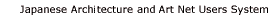koubai　勾配KEY WORD :　architecture / general termsIncline, slope, or pitch. Applied especially to the incline of the roof, yane koubai 屋根勾配, in traditional Japanese architecture. The roof pitch is determined by using the foundation as the base angle, i.e., the horizontal line. Then a right angle is formed by erecting an imaginary perpendicular, chuukou koubai 中鈎勾配. The measurements of the resulting right-angled triangle are used to determine the degree of pitch. For example： a) the pitch expressed as the angle between the hypotenuse and the base line is called kakudo koubai 角度勾配; b) the pitch expressed as the vertical measure divided by the horizontal measure (i.e.. the tan of the angle in (a) above) is called bunsuu koubai 分数勾配. For example, in figure 1, the bunsuu koubai is a/b, or tana. c) the pitch expressed as the ratio of the vertical measure against the horizontal, when the vertical is expressed in sun 寸 and the horizontal is taken as one shaku 尺, equivalent to 10 sun. This is called sunkoubai 寸勾配 or sunpou koubai 寸法勾配. For example, in figure 2, the incline is expressed as 4 sunkoubai. d) A 45 degree slope is known as *kanekoubai 矩勾配. e) the pitch expressed as the ratio of the vertical measure against the hypotenuse, when the vertical is expressed in sun 寸 and the hypotenuse is taken as one shaku. This is called *hirakoubai 平勾配. For example, in figure 3, the incline is expressed as 3 hirakoubai. f) Very steep inclines of more than 45 degrees are called kaeshikoubai 返し勾配 or norikoubai 乗り勾配. This is measure in one of two ways: (i) The angle in excess of 45 degrees is considered to be the kaeshikoubai. In figure 4, 45 degrees are subtracted, and a new right-angled triangle is drawn as shown. The pitch is then calculated as described in (e) above; in this case the kaeshikoubai is 3 sun because the hypotenuse is 1 shaku and the shorter side of the triangle is 3 sun. (ii) The kaeshikoubai is defined as the angle ABC in figure 5, using a version of the sun method described in (c) above, although this time the ratio is expressed as the horizontal measure against the vertical. The example in the diagram gives a kaeshikoubai of 4 sun , as the horizontal is 4 sun and the vertical, one shaku.REFERENCES:EXTERNAL LINKS:NOTES(C)2001 Japanese Architecture and Art Net Users System.　No reproduction or republication without written permission.